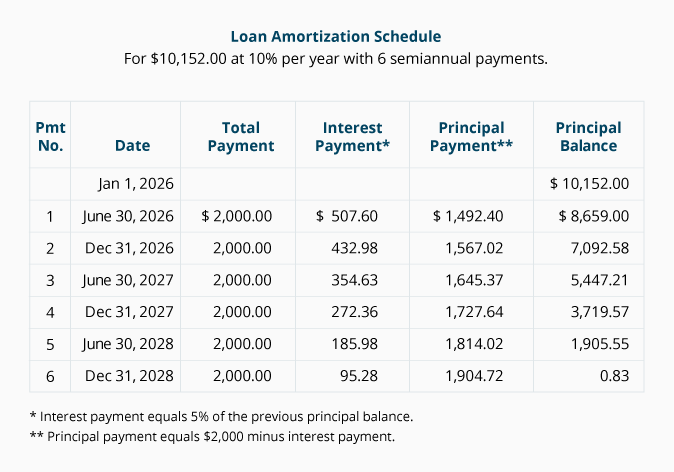# Calculating the Payment in an Ordinary Annuity (PMT)

Present value calculations allow us to determine the amount of the recurring payments in an ordinary annuity if we know the other components: present value, interest rate, and the length of the annuity. Exercises 5 and 6 will demonstrate how to solve for the payment amount.

### Exercise #5

On June 1, 2022, Grandma deposited \$1,733 into an account to help pay for Emily's summer volleyball camp for four consecutive years. The first camp is scheduled for June 2023. The account earns 6% interest per year, compounded annually. The interest earned on the account balance is deposited into the account on May 31 of each year. If Grandma wants the balance to be \$0 at the end of the four years, how much should she withdraw for Emily each June?

The following timeline helps us visualize the facts: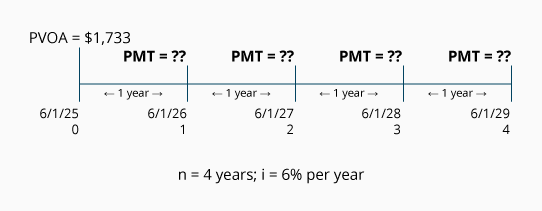Calculation of Exercise #5 using the PVOA Table

Using the above information and factors from our PVOA Table, we can solve for the unknown payment amount (PMT) as follows: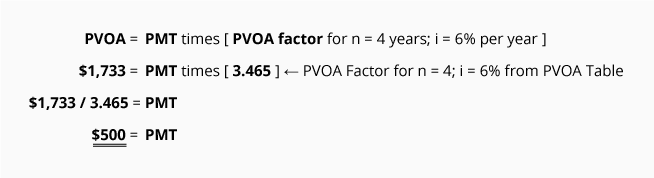We use simple algebra and the appropriate present value factor to determine that Grandma can withdraw \$500 each June 1 beginning in 2023.

The following table shows the account activity, confirming that \$500 can be withdrawn each year for four years: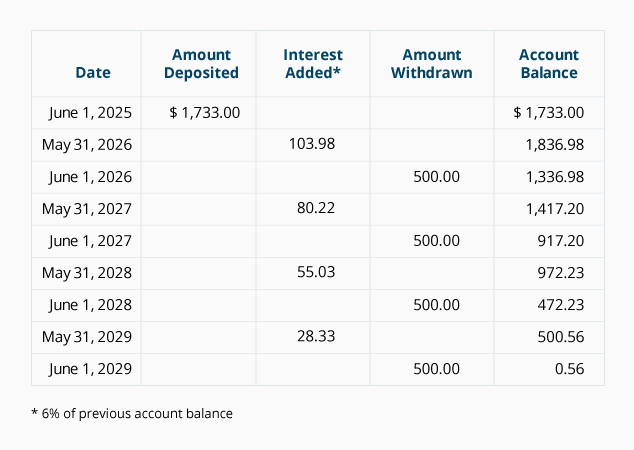### Exercise #6

Your company plans to borrow \$10,152 on January 1, 2023. You would like to repay the loan by making six semiannual loan payments beginning on June 30, 2023. The payments will be equal amounts and will cover a portion of both the interest (10% per year compounded semiannually) and the principal repayment. The payments will be paid on each June 30 and December 31. What will be the amount of each of the six payments?

Calculation of Exercise #6 using the PVOA Table

Our first step is to construct a timeline to organize the information: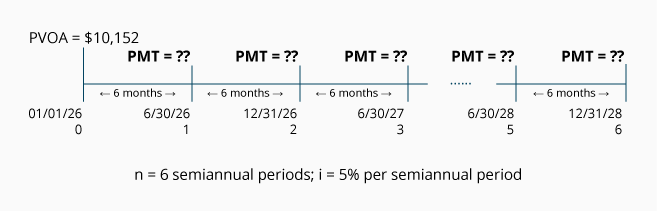Using the above information and factors from our PVOA Table, we can solve for the unknown payment amount (PMT) with the following equation: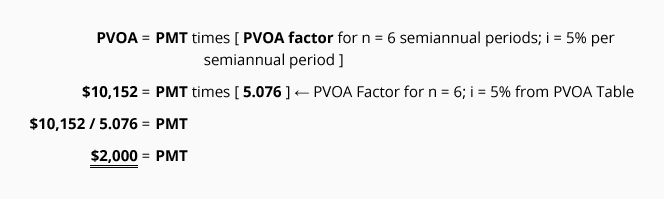We use simple algebra and the appropriate present value factor to determine that each of the six payments will be \$2,000. The first payment will be made on June 30, 2023 and the final payment will occur on December 31, 2025.

The following loan amortization schedule shows the amount of interest and principal contained in each loan payment and confirms that the loan will be paid by December 31, 2025.

Loan Amortization Schedule

(For \$10,152.00 at 10% per year with 6 semiannual payments.)